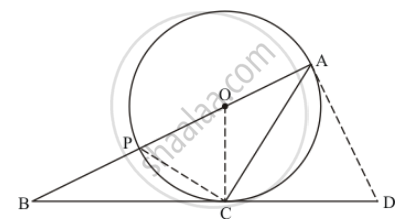Advertisement Remove all ads

# In the Given Figure, O is the Centre of the Circle and Bcd is Tangent to It at C. Prove that ∠Bac + ∠Acd = 90°. - Mathematics

Short Note

In the given figure, O is the centre of the circle and BCD is tangent to it at C. Prove that ∠BAC + ∠ACD = 90°.Advertisement Remove all ads

#### Solution

In the given figure, let us join D an A.Consider Δ OCA. We have,

OC = OA (Radii of the same circle)

We know that angles opposite to equal sides of a triangle will be equal. Therefore,

∠ OCA =∠ OAC  …… (1)

It is clear from the figure that

∠ DCA +∠ OCA=∠ OCD

Now from (1)

∠ DCA +∠OAC =∠ OCD

Now as BD is tangent therefore ∠ OCD=90^o

Therefore ∠ DCA +∠ OAC = 90^o

From the figure we can see that ∠ OAC =∠ BAC

∠ DAC + ∠ BAC = 90^o

Thus we have proved.

Is there an error in this question or solution?
Advertisement Remove all ads

#### APPEARS IN

RD Sharma Class 10 Maths
Chapter 8 Circles
Exercise 8.2 | Q 48 | Page 41
Advertisement Remove all ads
Advertisement Remove all ads
Share
Notifications

View all notifications

Forgot password?